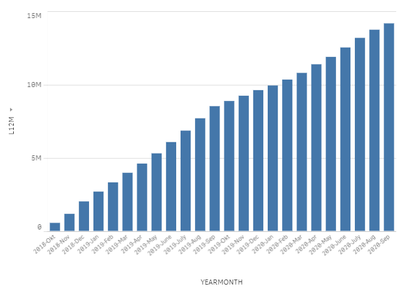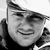# New to Qlik Sense

If you’re new to Qlik Sense, start with this Discussion Board and get up-to-speed quickly.

Announcements
QlikWorld 2023, a live, in-person thrill ride. Save \$300 before February 6: REGISTER NOW!
cancel
Showing results for
Did you mean:Contributor II

## Running Total in Bar Chart and only displaying last 12 months

Hi!

I'm trying to show a rolling 12 month sum for the latest 12 months, but the calculations are affected by the filtering of my Dimension axis to only display the last 12 months. I want the values to be unaffected by the choice of displaying last months only, so that the rolling sum for the last month still calculates the sum between month 12 and 23.

My current expression uses the Above function which I think causes the issue, since it's only using the displayed data and disregards months 13-23 in the calculation for month 12.

Dimension: MYDATECOLUMN.autoCalendar.YearMonth

Measure: RangeSum(Above( total Sum( {<[MYDATECOLUMN.autoCalendar.YearMonth] = >} MY_VALUES), 0, 12))

Output with last 12 months selected:Output with 24 months selected. Notice how values for 2019-Okt changes now because it has enough data in the graph to calculate rolling sum. I want these values for the last 12 months to be displayed in the above graph.I don't want to create a new table with the rolling sum as I need to be able to filter the results by user selections. I'm using Qlik Sense Enterprise in the web browser.

In short:

How can I create an expression which calculates a rolling 12 month sum, and only show the latest 12 months without affecting the L12M calculations?

Happy for any input!

Best regards,

Jakob

Labels (2)

• ### ROLLING SUM

1 Solution

Accepted SolutionsContributor II
Author

I think I got it figured out now. For anyone curious, I changed the expression to the following:

``````if([MYDATECOLUMN.autoCalendar.MonthsAgo]<13,
RangeSum( Above( total Sum( {\$<[MYDATECOLUMN.autoCalendar.YearMonth] = >} MY_VALUES) ,0, 12)))``````

and then unchecked "Include zero values" in the Data Handling tab. The dimension axis I left untouched, so as not to filter anything there. Now it displays the last 12 months only while still calculating the correct values for all months.

Output (note same values as the bigger graph above but only for the latest year)I will try and mark this thread as done. Ciao!

4 RepliesMVP

@flygandejakob  try below expression.  assuming [MYDATECOLUMN.autoCalendar.YearMonth]  is in YYYY-MMM format and not represented as text.

``=sum({<[MYDATECOLUMN.autoCalendar.YearMonth] ={">=\$(=date(addmonths(max([MYDATECOLUMN.autoCalendar.YearMonth] ),-11),'YYYY-MMM'))<=\$(=date(max([MYDATECOLUMN.autoCalendar.YearMonth] ),'YYYY-MMM'))"}>}aggr(rangesum(above(total sum({<[MYDATECOLUMN.autoCalendar.YearMonth] >}Sales),0,12)), ([MYDATECOLUMN.autoCalendar.YearMonth] ,(NUMERIC,ASCENDING))))``Contributor II
Author

I tried it but unfortunately it gives me the same values as before. Meaning the last 12 months for Oct-2019 only includes Oct-2019 whenever I filter the graph to show only Oct-2019 to Sep 2020. I wonder if there even is a way for QlikSense to calculate something it cannot see in the graph. My theory is that the above function can never be used here since there is no above when we filter so that Oct-2019 is the first displayed month

Or is the error perhaps in how I filter the Dimension axis?

``if(MYDATECOLUMN.autoCalendar.MonthsAgo <13,MYDATECOLUMN.autoCalendar.YearMonth)``Contributor II
Author

I think I got it figured out now. For anyone curious, I changed the expression to the following:

``````if([MYDATECOLUMN.autoCalendar.MonthsAgo]<13,
RangeSum( Above( total Sum( {\$<[MYDATECOLUMN.autoCalendar.YearMonth] = >} MY_VALUES) ,0, 12)))``````

and then unchecked "Include zero values" in the Data Handling tab. The dimension axis I left untouched, so as not to filter anything there. Now it displays the last 12 months only while still calculating the correct values for all months.

Output (note same values as the bigger graph above but only for the latest year)I will try and mark this thread as done. Ciao!MVP

Great that you found a solution to your problem. I have an alternative expression that should also do the trick.

sum( aggr( rangesum( above( total Sum( {\$<[MYDATECOLUMN.autoCalendar.YearMonth]=>} MY_VALUES),0,12)), MYDATECOLUMN.autoCalendar.YearMonth))Tags
Community Browser